Gradient Descent is an algorithm for online linear regression. In the simplest case, the algorithm predicts a sequence of numbers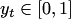. The prediction space is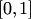. The master's prediction is the weighted average of the input vectors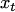. At each step the weights are updated by the rule, which depends on the gradient of a loss function: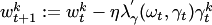.

For the bounded signal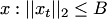and convex twice differentiable by the second argument loss function the Gradient Descent algorithm can achieve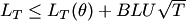,

where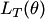is the loss of any linear function of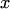, and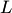is a bound for the derivative of the loss function: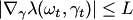. The best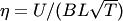. Here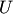us the norm of the experts: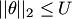The Gradient Descent algorithm was intestigated for this problem by Cesa-Bianchi et al. (1994), and is also known as Least Mean Squares algorithm for the square-loss function.

### Bibliography

• N. Cesa-Bianchi, P.M. Long, and M.K. Warmuth: Worst-case quadratic loss bounds for prediction using linear functions and gradient descent. IEEE Transactions on Neural Networks, 7(3):604-619, 1996.